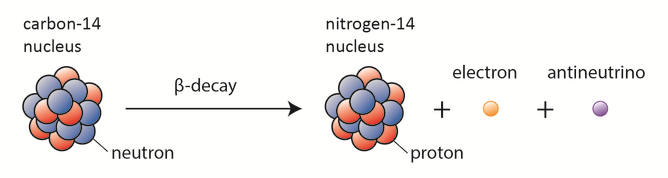## Beta-decay

In a typical beta decay, a radioactive nuclide emits a beta particle (an electron or positron) when a neutron/proton transforms into a proton/neutron (Beta minus, β−, decay/ Beta plus, β+, decay). This means that the atomic number of a parent nuclide will be increased or decreased by one unit, while the mass number remains unchanged. This mode of radioactive decay is of great importance in nuclear medicine and thus constitutes the crux of this research. The goal of this thesis is to adapt the properties of the emitted β− and β+ particles from 64Cu and 18F for a theoretical analysis and simulation using GEANT-4 tool kit.

## Interaction of Beta Particles with matter

To better detect beta radiation in several hundred KeVs range from 64Cu and 18F , it is important to understand how these beta particles interact with matter. Radiation in general deposits energy in matter through a series of ionizations and excitations. Understanding these interactions can provide the fundamental information for the development of portable nuclear imaging devices for efficient detection of beta particles in a feasible range of interest.

## Geant4 – Monte Carlo (MC) simulation

There are several MC programs available in nuclear imaging and internal dosimetry with many of them available as open source codes. Current review of those can be found in. There are packages that simulate the transport of radiation through matter such as: PENELOPE , GEANT4 , MCNP and EGS4. A number of tools for PET simulation have been developed over the years based on these codes, such as SIMSET , PETSIM or EIDOLON which are based on MCNP, and GATE on GEANT4 . A handful of these toolkits are limited by the complexity of the model, and the computation time required for simulation.
Although the theoretical analysis can give the average distance of beta particles traveling in matter, more detailed information can be acquired using GEANT4-MC simulation. Geant4 (GEometry ANd Tracking -4) is a simulation tool kit for the passage of particles through matter developed by the high energy physics community. Because of its original set of physics processes, modeling content and simulation accuracy, Geant4 toolkit is being used in studies in medical and space sciences as well as nuclear and accelerator physics.
Monte Carlo (MC) methods on the other hand, are numerical calculation methods based on random variable sampling. Named “Monte Carlo” by Von Neumann after the most well known center for gambling: The Monte Carlo in Monaco principality because of its similarity to the statistical simulations of the games of chance. The general idea of Monte Carlo analysis is to create a model, which is practically similar to the real physical system of interest, and to create interactions within that system based on known probabilities of occurrence using, whenever suitable, random sampling of Probability Density Functions (PDFs). Virtually, any complex system can in principle be modeled, if the distribution of events that occur in a system is known from experience or other means, and thus a PDF can be generated to simulate the real system.Interaction of Beta Particles with matter